Will

•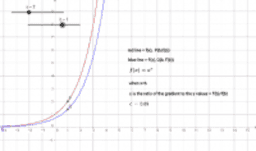Activity

Will

•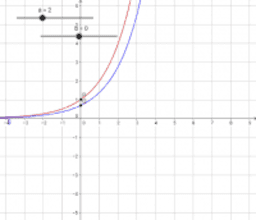Activity

Will

•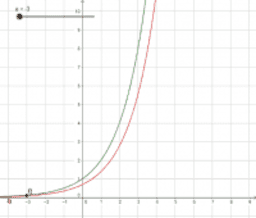Activity

Will

•spots

Activity

Will

•spots

Activity

Will

•perpendicular lines

Activity

Will

•perpendicular lines

Activity

Will

•perpendicular lines

Activity

Will

•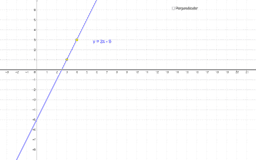Perpendicular lines

Activity

Will

•Activity

Will

•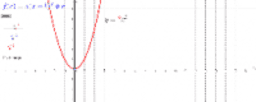Complete the square - investigating the graph

Activity

Will

•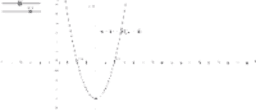Investigating roots of quadratic - factor form

Activity

Will

•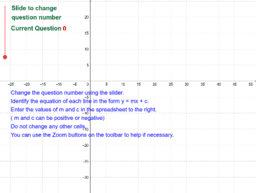Name the Line

Activity

Will

•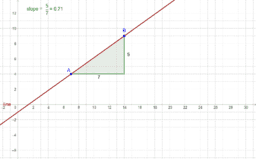Activity

Will

•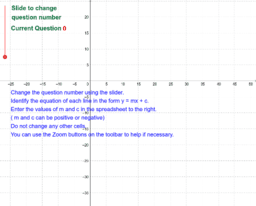Name the Line

Activity

Will

•Perpendicular lines

Activity

Will

•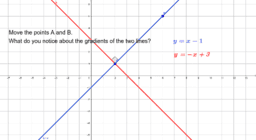Perpendicular lines

Activity

Will

•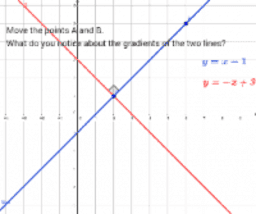Perpendicular lines

Activity

Will

•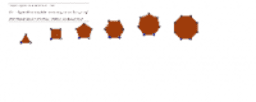Tesselations

Activity

Will

•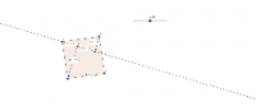Yue's geometry task - figure 3 (with lengths)

Activity

Will

•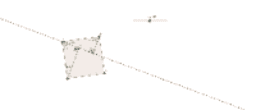Yue's geometry task - figure 2 (angles shown)

Activity

Will

•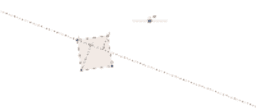Yue's geometry task - figure 1

Activity

Will

•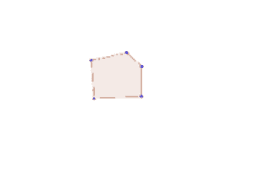Hello

Activity

Will

•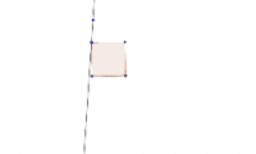Activity

Will